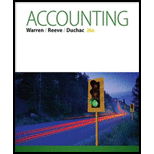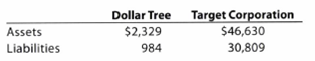Chapter 1, Problem 1.5EX### Accounting (Text Only)

26th Edition
Carl Warren + 2 others
ISBN: 9781285743615

#### Solutions

Chapter
Section### Accounting (Text Only)

26th Edition
Carl Warren + 2 others
ISBN: 9781285743615
Textbook Problem
1 views

# Accounting equationThe total assets and total liabilities (in millions) of Dollar Tree Inc. and Target Corporation follow:Determine the owners’ equity of each company.

To determine

Accounting equation:

Accounting equation is an accounting tool expressed in the form of equation, by creating a relation between resources or assets of a company and claims on the resources by the creditors and owners. Accounting equation is expressed as shown below:

Assets = Liabilities + Shareholders Equity

Owner’s equity of each company

Explanation

Calculate the Owner’s Equity of Company DT.

Assets =  Liabilities + Owners' EquityOwners' Equity =  Assets  Liabilities=$2,329$984=$1,345 Hence, the Owner’s Equity of Company DT is$1,345 million.

Calculate the Owner’s Equity of Corporation T

### Still sussing out bartleby?

Check out a sample textbook solution.

See a sample solution

#### The Solution to Your Study Problems

Bartleby provides explanations to thousands of textbook problems written by our experts, many with advanced degrees!

Get Started

#### Find more solutions based on key concepts